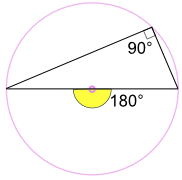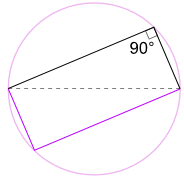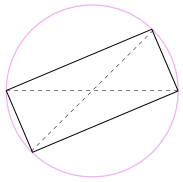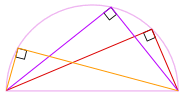# Circle Theorems

Some interesting things about angles and circles

## Inscribed Angle

First off, a definition:

Inscribed Angle: an angle made from points sitting on the circle's circumference.A and C are "end points"
B is the "apex point"

Play with it here:

images/circle-prop.js?mode=inscribe

When you move point "B", what happens to the angle?

## Inscribed Angle Theorems

Keeping the end points fixed ...

... the angle is always the same,
no matter where it is on the same arc between end points:(Called the Angles Subtended by Same Arc Theorem)

And an inscribed angle is half of the central angle 2a°(Called the Angle at the Center Theorem

Try it here (not always exact due to rounding):

images/circle-prop.js?mode=inscribe2

### Example: What is the size of Angle POQ? (O is circle's center)Angle POQ = 2 × Angle PRQ = 2 × 62° = 124°

### Example: What is the size of Angle CBX?Angle ADB = 32° also equals Angle ACB.

And Angle ACB also equals Angle XCB.

So in triangle BXC we know Angle BXC = 85°, and Angle XCB = 32°

Now use angles of a triangle add to 180° :

Angle CBX + Angle BXC + Angle XCB = 180°
Angle CBX + 85° + 32° = 180°
Angle CBX = 63°

## Angle in a Semicircle (Thales' Theorem)

An angle inscribed across a circle's diameter is always a right angle:(The end points are either end of a circle's diameter,
the apex point can be anywhere on the circumference.)

Play with it here:

images/circle-prop.js?mode=thales
 Why? Because: The inscribed angle 90° is half of the central angle 180° (Using "Angle at the Center Theorem" above)### Another Good Reason Why It WorksWe could also rotate the shape around 180° to make a rectangle!

It is a rectangle, because all sides are parallel, and both diagonals are equal.

And so its internal angles are all right angles (90°).

### Example: What is the size of Angle BAC?The Angle in the Semicircle Theorem tells us that Angle ACB = 90°

Now use angles of a triangle add to 180° to find Angle BAC:

Angle BAC + 55° + 90° = 180°
Angle BAC = 35°So there we go! No matter where that angle is
on the circumference, it is always 90°

### Finding a Circle's CenterWe can use this idea to find a circle's center:

• draw a right angle from anywhere on the circle's circumference, then draw the diameter where the two legs hit the circle
• do that again but for a different diameter

Where the diameters cross is the center!

### Drawing a Circle From 2 Opposite Points

When we know two opposite points on a circle we can draw that circle.

Put some pins or nails on those points and use a builder's square like this:A "Cyclic" Quadrilateral has every vertex on a circle's circumference:A Cyclic Quadrilateral's opposite angles add to 180°: a + c = 180° b + d = 180°### Example: What is the size of Angle WXY?Angle WZY + Angle WXY = 180°
69° + Angle WXY = 180°
Angle WXY = 111°## Tangent Angle

A tangent line just touches a circle at one point.

It always forms a right angle with the circle's radius.

1012, 3240, 1013, 3241, 1014, 3242, 1015, 3243, 1016, 3244